HOVGAARD PROBLEM

# 1HOVGAARD PROBLEM

## Problem definition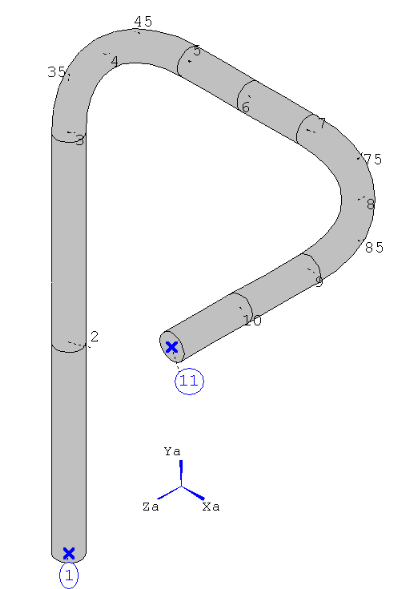Figure 2: ROHR2 MODEL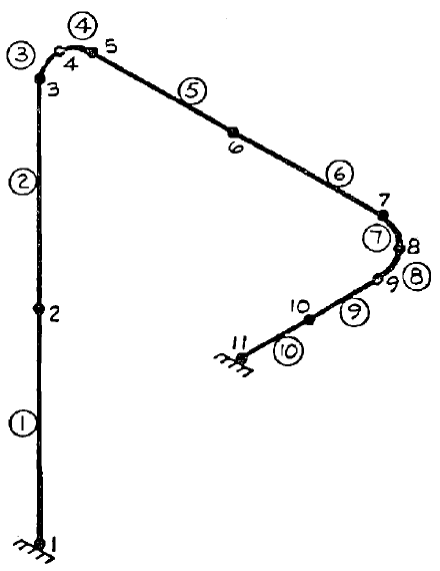Figure 1: NUREG MODEL

For the above system, verify the eigenfrequencies, mode shapes and deflections due to a given excitation.

## References (NUREG)

P.Bezler/ M. Hartzman/ M. Reich, Piping Benchmark Problems, Vol. 1,

Dynamic Analysis Uniform Support Motion; Response Spectrum Method,

Division of Technical Information and Document Control, U.S. Nuclear Regulatory Commission, Washington D.C., 1980, Chapter 3.1, pg. 15; Chapter 4.1, pg. 23-47

This problem is a simple, three-dimensional piping system made up of only straight line and bend pipe elements between two fixed anchors (node 1; 11) see figure Figure 1.

The computation of bend element flexibility factors assumed that there is no internal pressure. The system has no distributed mass (no internal generation of element mass). All masses are input as lumped masses. For the solution only five frequencies were calculated. A single input spectrum, shown in Figure , is applied with the weighting factors' of 1.0, 0.667 and 1.0 in the X, Y and Z global directions respectively. As all resultant system natural frequencies are spaced greater than 10% apart, clustering does not occur and the solution is therefore independent of the combination sequence employed. This problem was selected as a benchmark because its simplicity allows the checking of key results.

Model data:

 Variable Description Unit Used Value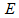Modulus of Elasticity lbs/inchÂ² 24000000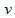Poisson's ratio --- 0.3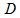Outside Diameter inch 7.288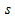Wall thickness inch 0.241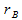Bend radius inch 36.3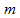Mass of several nodes lb ---

Table 1: Overview of the used variables

The system is submitted to the following response spectrum: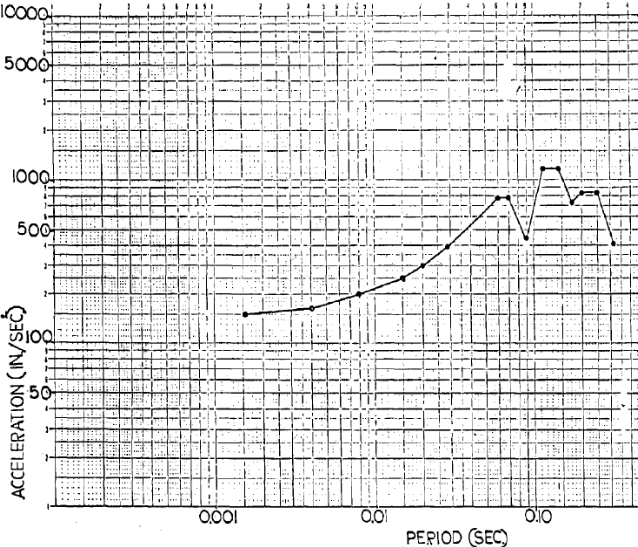Figure 3: Response spectrum

## Model description (ROHR2)

The coordinates of the nodes in the EPIPE input file are given in inch. They are converted to feet for the ROHR2 input: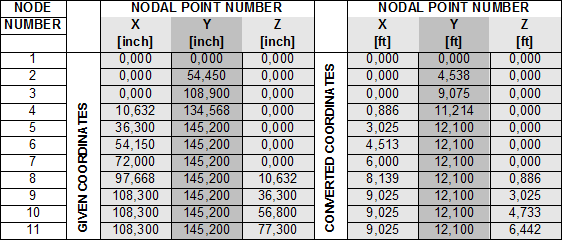Table 2: NODAL POINT NUMBER

The masses of the nodes in the EPIPE input file are given in slinch. They are converted to pound for the ROHR2 input: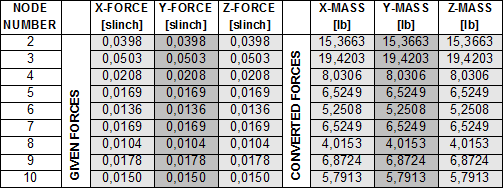A load case â€œEigenvalues was created to calculate the first 5 mode shapes.

In addition a load case â€œdynamic earthquakeâ€ was generated to apply the requested response spectra. The superposition was set to SRSS for the mode shapes.

## Result comparisons

The following five frequencies are listed in the results-output of the EPIPE-documentation.

 Value No Reference NUREG [1/sec] ROHR2 [1/sec] Difference [%]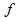1 28.535 28.530 <0.02 2 55.772 55.811 <0.07 3 81.500 81.414 <0.11 4 141.742 141.755 <0.01 5 162.820 163.236 <0.26

Table 1: Comparison of the resulting frequencies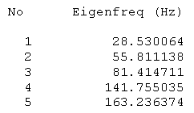Table 2: Eigenfrequencies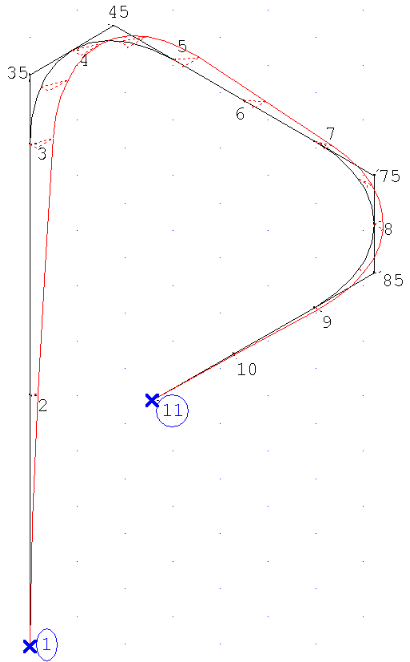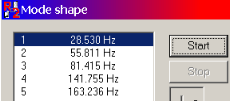### Deflections of the first Eigenvalue

The figures an tables below compare only two points of each mode shape. All results are given in the global coordinate system. The defections of the mode shapes can be scaled to any arbitrary value. In the reference calculation the scaling was based on a generalized mass of 1.0 slinch inÂ².

In the ROHR2 model the generalized mass is automatically chosen as 0.009369586 slinch inÂ².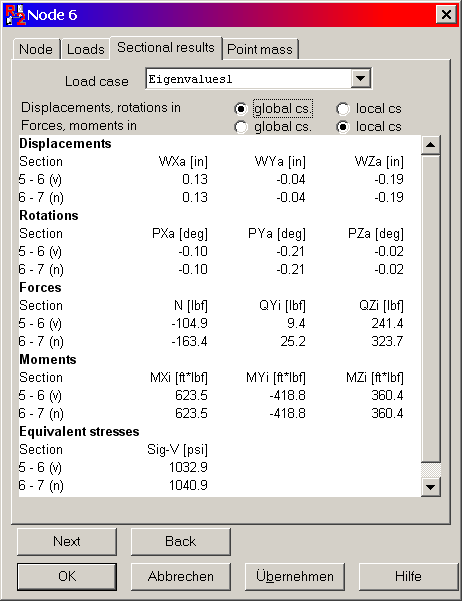Value Æ’ [1/sec] Reference NUREG mass= 1 slinch inÂ² [inch] ROHR2 mass= 9,36E-3 slinch inÂ² [inch] ROHR2 gmass= 1 slinch inÂ² [inch] Difference [%]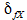28.530 1.38 0.13 1.34 <3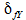-0.37 -0.04 -0.41 <11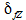-1.94 -0.19 -1.96 <2

Table 3: Comparison of the deflection of node 6

### Deflections of the second Eigenvalue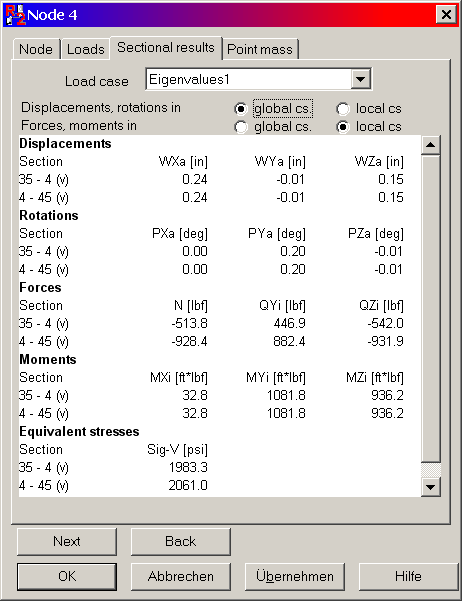Value Æ’ [1/sec] Reference NUREG mass= 1 slinch inÂ² [inch] ROHR2 mass= 9,36E-3 slinch inÂ² [inch] ROHR2 mass =1 slinch inÂ² [inch] Difference [%]55.811 2.28 0.24 2.27 <1-0.01 -0.01 -0.10 <-1.57 0.15 0.155 <2

### Deflections of the third Eigenvalue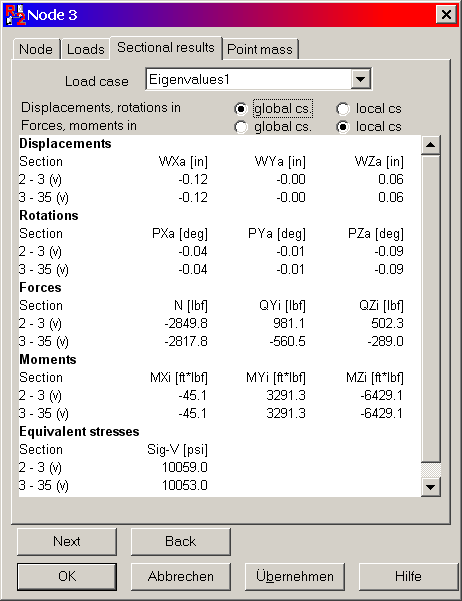Value Æ’ [1/sec] Reference NUREG mass= 1 slinch inÂ² [inch] ROHR2 mass= 9,36E-3 slinch inÂ² [inch] ROHR2 mass =1 slinch inÂ² [inch] Difference [%]81.415 -1.21 -0.12 -1.24 <3-0.02 -0.00 -0.00 -0.62 0.06 0.62 <1

### Deflections of the fourth Eigenvalue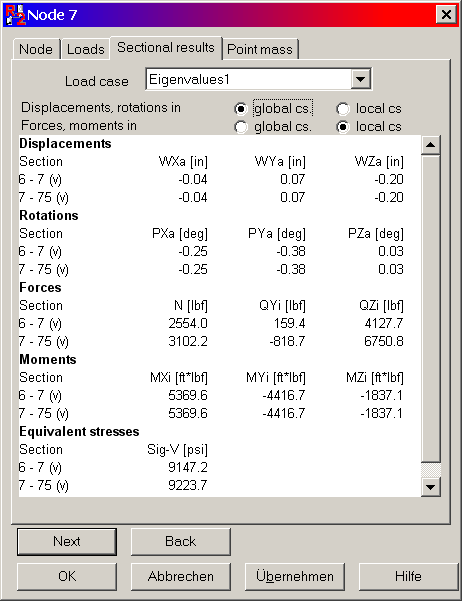Value Æ’ [1/sec] Reference NUREG mass= 1 slinch inÂ² [inch] ROHR2 mass= 9,36E-3 slinch inÂ² [inch] ROHR2 mass =1 slinch inÂ² [inch] Difference [%]141.755 0.42 -0.04 -0.41 <3-0.75 0.07 0.72 <42.02 -0.20 -2.01 <1

### Deflection of the fifth Eigenvalue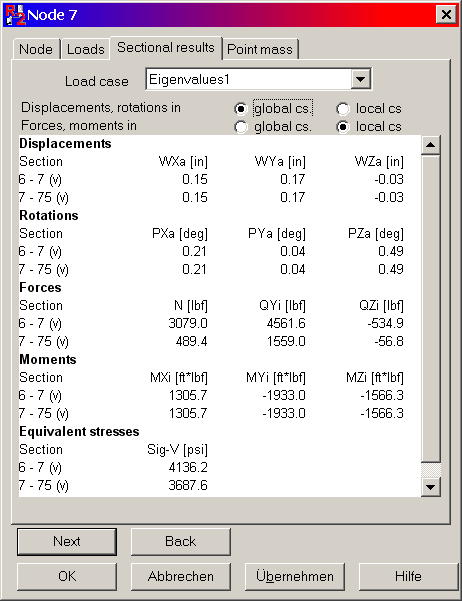Value Æ’ [1/sec] Reference NUREG mass= 1 slinch inÂ² [inch] ROHR2 mass= 9,36E-3 slinch inÂ² [inch] ROHR2 mass =1 slinch inÂ² [inch] Difference [%]163.236 1.51 0.15 1.55 <31.73 0.17 1.76 <2-0.28 -0.03 -0.31 <11

### Participation Factors

The figures an tables below compare the Participation Factors. All results are given in the global coordinate system. The Participation Factors of the mode shapes can be scaled to any arbitrary value. In the reference calculation the scaling was based on a generalized mass of 1.0 slinch inÂ².

In the ROHR2 model the generalized mass is automatically chosen as 0.009369586 slinch inÂ².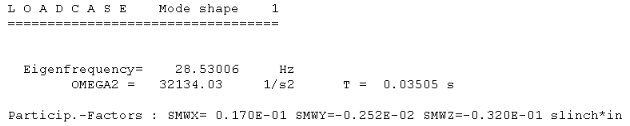Value Æ’ [1/sec] Reference NUREG gmass= 1 slinch inÂ² [slinch in] ROHR2 gmass= 9,36E-3 slinch inÂ² [slinch in] ROHR2 gmass= 1 slinch inÂ² [slinch in] Difference [%] SMWX 28.530 0.175 0.017 0.175 <1 SMWY -0.025 -0.002 -0.025 <1 SMWZ -0.331 -0.032 -0.331 <1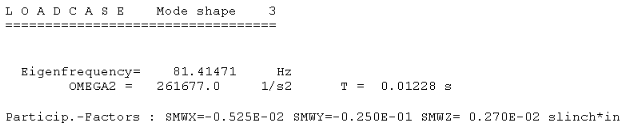Value Æ’ [1/sec] Reference NUREG gmass= 1 slinch inÂ² [slinch in] ROHR2 gmass= 9,36E-3 slinch inÂ² [slinch in] ROHR2 gmass= 1 slinch inÂ² [slinch in] Difference [%] SMWX 81.415 -0.053 -0.005 -0.054 <2 SMWY -0.258 -0.025 -0.258 <1 SMWZ 0.028 0.002 0.028 <1

### Node Displacements

(direction factors 1000, 666, 1000)

Point 4

 Value Reference NUREG [in] ROHR2 [in] ROHR2 [in] Difference [%] WXa 7.41E-3 7.52 7.52E-3 <2 WYa 6.12E-4 0.62 6.20E-4 <2 WZa 1.74E-2 17.69 1.77E-2 <2

Point 8

 Value Reference NUREG [in] ROHR2 [in] ROHR2 [in] Difference [%] WXa 5.82E-3 5.90 5.90E-3 <2 WYa 1.99E-3 2.02 2.02E-3 <2 WZa 1.68E-3 1.70 1.70E-3 <2

## Conclusion

The results are generally close to the references by the NUREG-example (benchmark problems 1, Hovgaard problem). There are several reasons for minor differences.

The first one results of the scale factor in ROHR2, which is different to EPIPE. The reference-program used a unity-matrix to calculate the deflections of each node. This matrix has a constant value of 1 tmÂ². In ROHR2 it is used a alternative method scale the deflections. It calculates a generalized mass, which generate displacement which are easy to interpret. The following value was used:

In order to compare the deflections between NUREG and ROHR2, it is necessary to multiply the EPIPE-results by the generalized mass of mode shape. The second inaccuracy of the results is shown at point 4 (Load case Eigenvalue; second Eigenvalue; deflection Î´fY). If the result is relatively small (Î´fY= 0,0059 inch), the relative difference will become bigger, though the difference of the displacement vector length and direction is actually negligible.

## Files

Problem1_0.r2w

SIGMA Ingenieurgesellschaft mbH www.rohr2.com